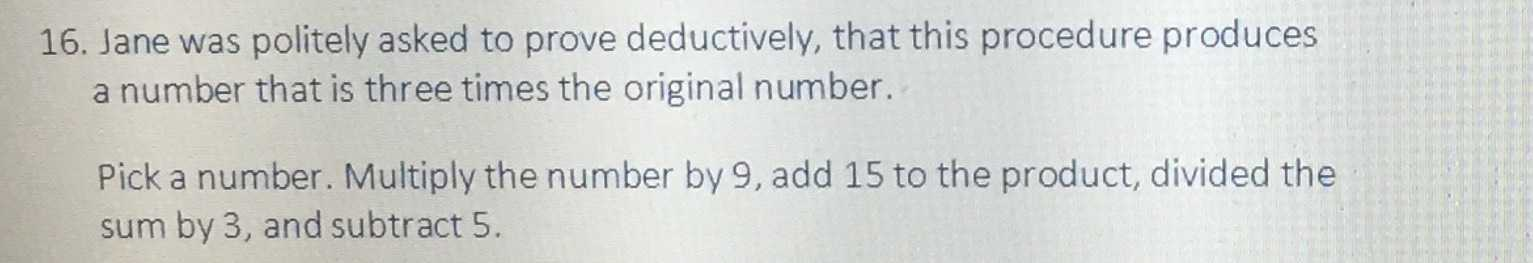### ¿Todavía tienes preguntas de matemáticas?

Pregunte a nuestros tutores expertos
Algebra
Pregunta16. Jane was politely asked to prove deductively, that this procedure produces a number that is three times the original number. Pick a number. Multiply the number by $$9$$ , add $$15$$ to the product, divided the sum by $$3$$ , and subtract $$5$$ .